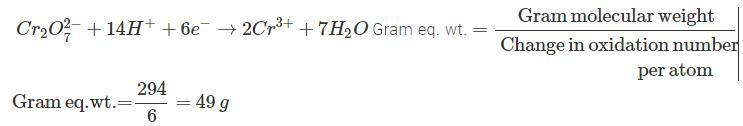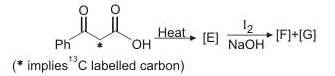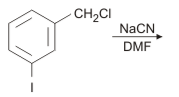Courses

# JEE(MAIN) Chemistry Mock Test - 5

## 30 Questions MCQ Test JEE Main Mock Test Series 2020 & Previous Year Papers | JEE(MAIN) Chemistry Mock Test - 5

Description
This mock test of JEE(MAIN) Chemistry Mock Test - 5 for JEE helps you for every JEE entrance exam. This contains 30 Multiple Choice Questions for JEE JEE(MAIN) Chemistry Mock Test - 5 (mcq) to study with solutions a complete question bank. The solved questions answers in this JEE(MAIN) Chemistry Mock Test - 5 quiz give you a good mix of easy questions and tough questions. JEE students definitely take this JEE(MAIN) Chemistry Mock Test - 5 exercise for a better result in the exam. You can find other JEE(MAIN) Chemistry Mock Test - 5 extra questions, long questions & short questions for JEE on EduRev as well by searching above.
QUESTION: 1

Solution:
QUESTION: 2

Solution:
QUESTION: 3

### Which of the following sequence is correct as per Aufbau principle?

Solution:
QUESTION: 4
Which of the following is the least polar molecule?
Solution:
QUESTION: 5
Which of the following alkyl group has the maximum + I effect?
Solution:
QUESTION: 6
Consider the ground state of Cr atom (Z = 24). The numbers of electrons with the azimuthal quantum numbers, l = 1 and 2 are, respectively :
Solution:
QUESTION: 7
Alkyl groups are o- and p-directing because of
Solution:
QUESTION: 8
Which of the following factors do not favour electrovalency?
Solution:
QUESTION: 9
Glucose reacts with acetic anhydride to form
Solution:
QUESTION: 10
Which of the following shows geometrical isomerism ?
Solution:
QUESTION: 11
The co-ordination no. of a metal in a co-ordination compounds is
Solution:
QUESTION: 12
Four successive members of the first row transition elements are listed below with their atomic no. Which of them is expected to have the highest third ionisation enthalpy?
Solution:
QUESTION: 13

Which is a planar molecule?

Solution:
QUESTION: 14
In decinormal solution, CH₃COOH acid is ionised to the extent of 1.3%. If log 1.3=0.11. What is the pH of the solution?
Solution:
The dissociation of acetic acid is:

CH3COOH →  CH3COO- + H+

c – cα  cα

Concentration = 0.1 M

Degree of ionization, α = 1.3 %

c α = (0.1) * (1.3* 10^-2)

[H +]=1.3 * 10^-3

pH can be determined as:

-log (H^+) = pH = - log (1.3 * 10^-3) = 2.87

Thus the pH of the solution is 2.87
QUESTION: 15

Sodium nitroprusside reacts with sulphide ion to give a purple colour due to the formation of :

Solution:
QUESTION: 16
Which are isomers?
Solution: Isomers are molecules that have the same molecular formula, but have a different arrangement of the atoms in space. That excludes any different arrangements which are simply due to the molecule rotating as a whole, or rotating about particular bonds. For example, both of the following are the same molecule.
QUESTION: 17
Chlorination of toluene with excess of chlorine in the presence of light and heat followed by treatment with aqueous NaOH gives
Solution:
QUESTION: 18

In a polymer 30% molecules have molecular weight 20,000, 40% molecules have molecular weight 30,000 and the rest 30% molecules have molecular weight 60000. The weight average molecular weight of the polymer is

Solution:
QUESTION: 19
Electrolysis of molten sodium chloride leads to the formation of
Solution:
QUESTION: 20
The elements, which occupy the peaks of ionisation energy curve, are
Solution: In the periodic table, noble gases have highest ionisation enthalpies and hence are chemically inert. Thus, they occupy the peaks of ionisation energy curve.
QUESTION: 21
In acidic medium dichromate ion oxidizes ferrous ion to ferric ion. If the gram molecular weight of potassium dichromate is 294 grams, its gram equivalent weight is....grams.
Solution:QUESTION: 22
How many monobromo isomers, including stereoisomers could be formed in the reaction of 2, 2 - dimethyl butane with bromine in presence of light?
Solution:
QUESTION: 23
Adsorption due to strong chemical forces is called
Solution:
QUESTION: 24
When calomel reacts with ammonium hydroxide, we get
Solution:
QUESTION: 25

In the following reaction sequence, the correct structures of E, F and G areSolution:
QUESTION: 26

Among the following metal carbonyls, the C ― O bond order is lowest in

Solution:

An anionic carbonyl complex can delocalise more electron density to antibonding π - orbital (dπ - pπ back -bonding) of CO and thus lower the bond order.

QUESTION: 27

A positron is emited from 2311Na . The ratio of the atomic mass and atomic number of the resulting nuclide is

Solution:
QUESTION: 28

The species having bond order different from that in CO is

Solution:
QUESTION: 29

On the basis of the following thermochemical data:
Δ f G0 H+ aq = 0 .
H2O(l)  → H+ (aq) + OH- (aq)   ; Δ H = 57.32 k J
H2 g + 1/2 O2 g → H2O(l)  ; Δ H = − 286.2 k J
The value of enthalpy of formation of OH - ion at 250C is

Solution:
QUESTION: 30The main product in the above reaction is

Solution: